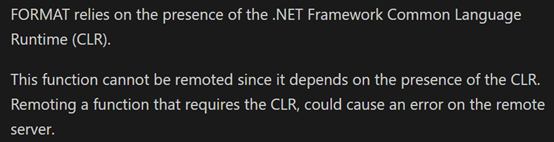### [SQL Server] Tiny format's problem

SQL2012推出format function，這項加強，對於開發人員，在處理跨國系統日期和時間顯示相當方便。

BOL推薦一般資料類型轉換，請使用cast或convert，日期和時間處理，主要就是format function工作，``````reate TABLE #result
(
i INT,
ms decimal(10,2)
);
go
declare @i int = 1, @x int, @j int = 10000;
declare @t0 datetimeoffset(7)=SYSDATETIMEOFFSET(), @t1 datetime2, @t2 datetime;
while @i<=5
begin
SELECT @x = 1, @t1 = SYSDATETIME();
while @x<=@j
begin
if @i=1
set @t2= CONVERT(CHAR(8), @t0, 112);
else if @i=2
set @t2 = CONVERT(DATE, @t0);
else if @i=3
set @t2 = CAST(@t0 AS DATE);
else if @i=4
set @t2 = CONVERT(DATE, FORMAT(@t0, N'yyyy-MM-dd'));
else if @i=5
set @t2 = FORMAT(@t0, N'yyyy-MM-dd');

set @x+=1;
end
INSERT #result with(tablock)
SELECT @i, DATEDIFF(MILLISECOND, @t1, SYSDATETIME());
set @i+=1;
end
GO 10

SELECT
i, method =
case
when i=1 then 'CONVERT(CHAR(8), @t0, 112)'
when i=2 then 'CONVERT(DATE, @t0)'
when i=3 then 'CAST(@t0 AS DATE)'
when i=4 then 'CONVERT(DATET, FORMAT(@t0, yyyy-MM-dd))'
else 'FORMAT(@t0, yyyy-MM-dd)'
end,
MIN=MIN(ms), MAX=MAX(ms), AVG=AVG(ms)
FROM #result
GROUP BY i
ORDER BY i;

drop table #result
``````可以看到method 1和method 4/5差距約4~5倍，

FORMAT (Transact-SQL)

SQL Server v.Next (Denali) : CTP3 T-SQL Enhancements : FORMAT()

[SQL SERVER][Memo]全時區轉換